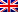# Seminario di Analisi Matematica

Mercoledì 18 maggio 2022 alle ore 14:30, Antonio J. Fernandez (ICMAT - Madrid) terrà il Seminario di Analisi Matematica dal titolo "Singular limits for a half-Laplacian Liouville type equation."

Abstract: We consider the nonlocal Liouville type equation
(-Delta)^(1/2)u = epsilon * k(x) e^u, u > 0 in I
u = 0 in R \ I
where I is a union of d >= 2 disjoint bounded intervals, k is a smooth bounded function with positive infimum and epsilon > 0 is a small parameter. For any integer 1 <= m <= d, we construct a family of solution u_\epsilon which blow-up at m distinct points of I and for which epsilon *(integral of k(x) e^u) --> 2m*pi as epsilon --> 0\$. Moreover, we show that, when d = 2 and m is suitably large, no such construction is possible. The talk is based on a joint work with Matteo Cozzi (Milano, Italy).

Il seminario avrà luogo in presenza presso il Dipartimento di Matematica e Fisica, L.go S. L. Murialdo 1 - Aula 211

This post is also available in: Link identifier #identifier__170806-5Eng